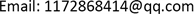1. 引言

{ d x d t = x ( a 1 − b 1 x − c 1 y ) d y d t = y ( a 2 − b 2 y + c 2 x )

{ d s d t = ( b − θ r H K ) H − ( d + ( 1 − θ ) r H K ) s − β s i d i d t = β s i − ( d + ( 1 − θ ) r H K ) i − m i y α + i d y d t = ( ε m i α + i − e ) y (1.1)

2. 模型改进

{ d s d t = ( b − θ r H K ) H − ( d + ( 1 − θ ) r H K ) s − β s i − m s y α + H d i d t = β s i − ( d + ( 1 − θ ) r H K ) i − m i y α + H d y d t = ( ε m H α + H − e ) y (2.1)

3. 平衡点及稳定性3.1. 平衡点

3.2. 局部稳定性

J E 1 = ( − r d − r θ − K β − m K α + K 0 K β − ( d + ( 1 − θ ) r ) 0 0 0 ε m K α + K − e ) (3.1)

J E 2 = ( − ( θ r + ( 1 − θ ) r s 2 K + β i 2 ) b − 2 r θ − ( 1 − θ ) r s 2 K − β s 2 − m s 2 α + K β i 2 − ( 1 − θ ) r i 2 K β s 2 − d − ( 1 − θ ) r − ( 1 − θ ) r K − m i 2 α + K 0 0 ε m K α + K − e ) (3.2)

J ˜ E 2 = ( − ( θ r + ( 1 − θ ) r s 2 K + β i 2 ) b − 2 r θ − ( 1 − θ ) r s 2 K − β s 2 β i 2 − ( 1 − θ ) r i 2 K β s 2 − d − ( 1 − θ ) r − ( 1 − θ ) r K ) (3.3)

3.3. 全局稳定性

i ≥ C e ( K β − ( 1 − θ ) r − d ) t   (3.4)

M = { ( s , i , y ) : V ˙ = 0 } = { i = 0 } (3.5)

{ d s d t = r s ( 1 − s K ) − m s y α + s d y d t = ( ε m s α + s − e ) y (3.6)

{ d H d t = r H ( 1 − H K ) d i d t = β s i − ( d + ( 1 − θ ) r H K ) i (3.7)

{ d H d t = r H ( 1 − H K ) d x d t = − ( d + r ) x + ( β + r H K ) H x − β H x 2 (3.8)

{ d H d t = r H ( 1 − H K ) − m H y α + H d i d t = β s i − ( d + ( 1 − θ ) r H K ) i − m i y α + H d y d t = ( ε m H α + H − e ) y (3.9)

{ d H d t = r H ( 1 − H K ) − m H y α + H d i d t = β s i − ( d + ( 1 − θ ) r H K ) i − m i y α + H (3.10)

3.4. 正平衡点及极限环

{ d H d t = r H ( 1 − H K ) − m H y α + H d y d t = ( ε m H α + H − e ) y (3.11)

，且

，即时，方程(3.16)存在唯一正根；此时，系统(2.1)有唯一的正平衡点，其中。由定理3.1的证明过程可知，

4. 结论

 赵姣, 魏春金, 王宝童, 张树文. 疾病在食饵中传播的捕食–食饵模型[J]. 生物数学学报, 2015(4): 709-713.

 Lasalle, J.P. (1968) Stability Theory for Ordinary Differential Equations. Journal of Differential Equations, 4, 57-65. https://doi.org/10.1016/0022-0396(68)90048-X

 陈兰荪, 井竹君. 捕食者–食饵相互作用中微分方程的极限环存在性和唯一性[J]. 科学通报, 1984, 29(9): 521-523.

 马知恩, 周义仓, 李承治. 常微分方程定性与稳定性方法[M]. 第二版. 北京: 科学出版社, 2015.

References赵姣, 魏春金, 王宝童, 张树文. 疾病在食饵中传播的捕食–食饵模型[J]. 生物数学学报, 2015(4): 709-713.Lasalle, J.P. (1968) Stability Theory for Ordinary Differential Equations. Journal of Differential Equations, 4, 57-65. <br>https://doi.org/10.1016/0022-0396(68)90048-X陈兰荪, 井竹君. 捕食者–食饵相互作用中微分方程的极限环存在性和唯一性[J]. 科学通报, 1984, 29(9): 521-523.马知恩, 周义仓, 李承治. 常微分方程定性与稳定性方法[M]. 第二版. 北京: 科学出版社, 2015.